# 13 Interesting Facts About Geometry

Geometry is something which makes us discover patterns, finds lengths, breadth, areas, angles and in short, make our understanding better when it comes to shapes and sizes and the world around us.1. Origin of Geometry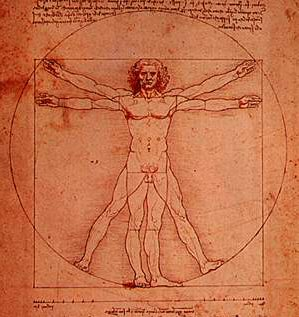The story of the origin of the word “Geometry” makes up an interesting piece. It comes from a Greek word- ‘Geo’ meaning ‘Earth’ and ‘Metria’ meaning ‘Measure’. So basically it is the measurement of Earth.
Source: wikipedia, Image: ancientmaths.com

2. Father of GeometryGreek Mathematician, Euclid did some amazing works in geometry that includes the influential “Elements”, which was part of text books for teaching mathematics until round the early 20th century. Euclid lived around the years of 300BC and because of his contribution, he is known as “Father of Geometry”.
Source: wikipedia, Image: wikipedia

3. History of Geometry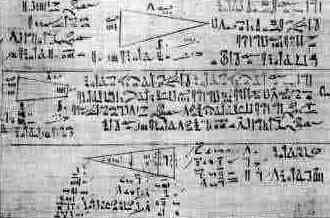The use of Geometry principles dates back to 3000 BC where Ancient Egyptians used various geometric equations to calculate area of circles among other formulas.
Source: wikipedia, Image: history.com

4. Pythagoras Theorem is OLDThe only theorem which we remember out of all the complicated geometry is a Pythagoras theorem, relating to the three sides of a right angle triangle: a² + b² = c². You will be surprised to know that this theorem was made by Greek philosopher and mathematician who lived around the year of 500 BC.
Source: wikipedia

5. Other Mathematicians contribution to GeometryAnother famous mathematician Archimedes of Syracuse of 250 BC played an important role in workings of geometry. He worked towards determining the volume of objects with irregular shapes.
Source: mathsisfun.com

6. Greeks used Geometry in making Building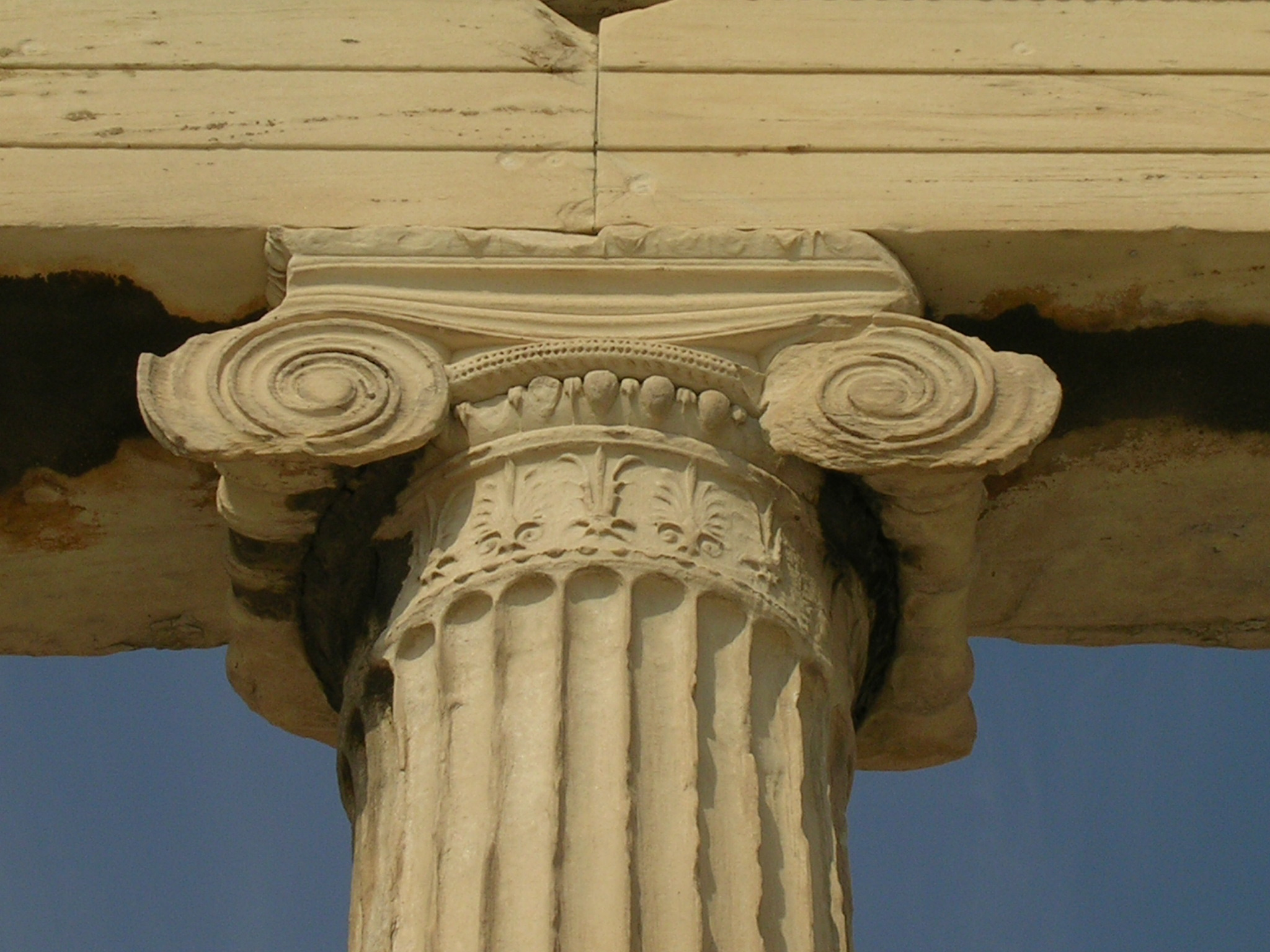Greeks were so keen for using Geometry that they made artwork and leasing buildings based on golden ration of approximately 1.618. Now you have another reason to love this subject!
Source: geometrymaths.weebly.com, Image: architecture.eu

7. The Powerful tools of Geometry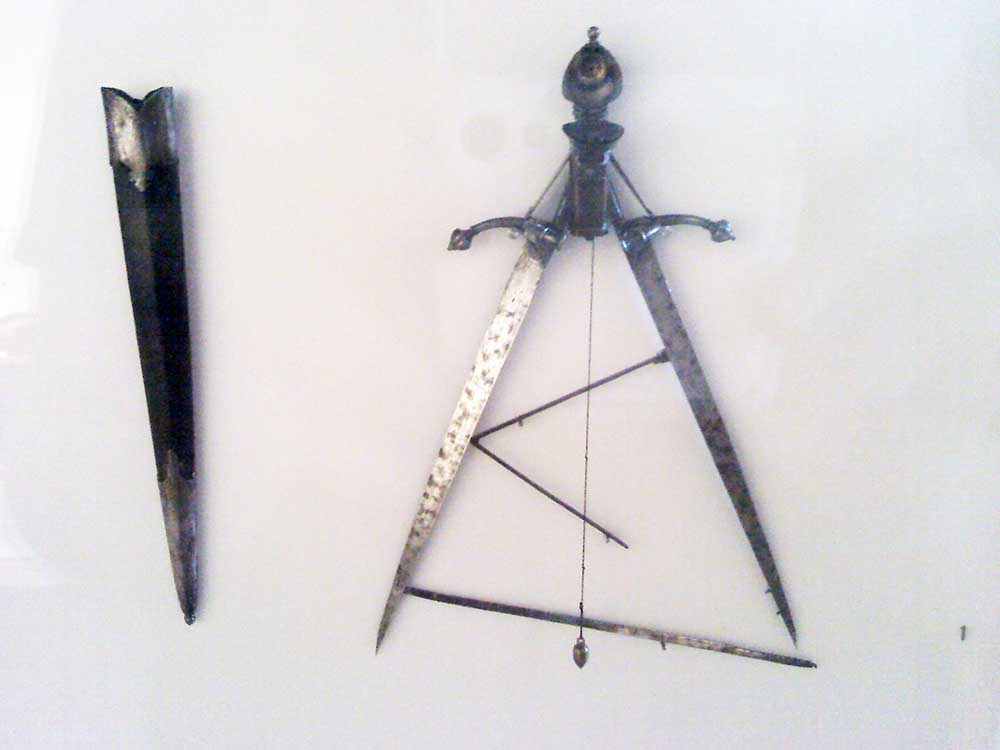Two of the most powerful tools of geometry which helped in the advancement of subject which helped in construction of various lengths, angles and geometric shapes were Compass and Straight edge.
Source: oureverydaylife.com, Image: flickr

8. Babylonians and Pi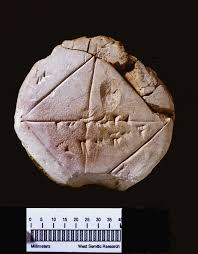It is believed that Babylonians in the ancient era came up with the measurement of circle which was approximately 3 times of the diameter. Interestingly it is quite close to today’s measurement of Pi (around 3.14)
Source: wikipedia, Image: mathsisfun.com

9. Branches of Geometry

Bet when we take Geometry classes, we hardly think it has so many branches to study from. Apparently this subject is very diverse with many branches like Euclidean Geometry, Analytic, Projective, Differential, Topology, Non- Euclidean.
Source: wikipedia

10. The Fascinating Eucler’s Formula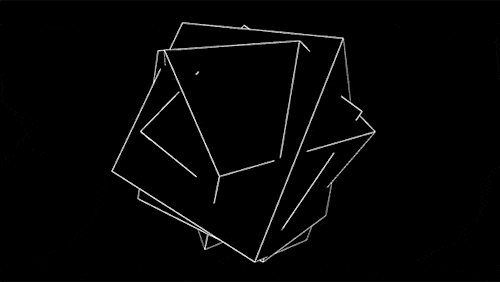If we take any three dimensional solid with flat faces known as polyhedron- for instance a cube, pyramid or a soccer ball, then adding the number of faces to number of vertices and then subsequently subtracting the no. of edges always give us the answer of 2. E.g. Cube possessing 6 faces, 8 vertices and 12 edges would come to 6+8-12= 2.
Source: wikipedia

11. Geometry is necessary for Computers and CalculatorsThe modern day geometry has come up a long way in its development stages and is used for many areas like raw computing power of today’s computer. Knowledge of this subject is important for computer graphics or calculator to solve structural problems.
Source: mathsisfun.com, Image: digital.artnetwork.com

12. The Early Phase of Geometry EraThe beginning of geometry was discovered by people in ancient Indus Valley and ancient Babylonia from 3000BC. It was discovered for practical purposes of construction, astronomy, surveying and various different crafts. Egyptians were also part of the early phase of Geometry Era.
Source: wikipedia, Image: ancientcultures.co.in

13. Introduction of 3 Dimensional GeometryIn Renaissance period of Projective Geometry, artists like Da Vinci and Durer discovered methods to represent 3D objects on 23 surfaces. These were refined in the 19th and 20th century and in 20th century, projective geometry was used for computer graphics.
Source: geometrymaths.weebly.com, Image: progressive.regressive.com